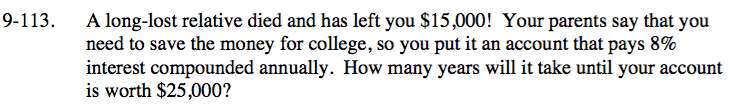### Home > INT3 > Chapter Ch9 > Lesson 9.2.2 > Problem9-113

9-113.

A long-lost relative died and has left you $15,000! Your parents say that you need to save the money for college, so you put it an account that pays 8% interest compounded annually. How many years will it take until your account is worth$25,000? Homework Help ✎This is an exponential function of the form y = abx, where a is the initial value, b is the multiplier, x is the number of years, and y is the amount of money saved.

Substitute the values given into the equation.

Divide both sides by 15000.

Remember the Power Property of Logarithms and take the log of both sides.

Solve for t.

The account will be worth 25000 in between 6 and 7 years.

25000 = 15000(1.08)t

$\frac{5}{3} = (1.08)^t$

$\text{log}\left(\frac{5}{3}\right) = t(\text{log}(1.08))$

t = 6.64